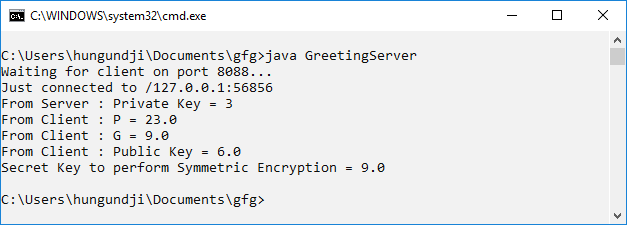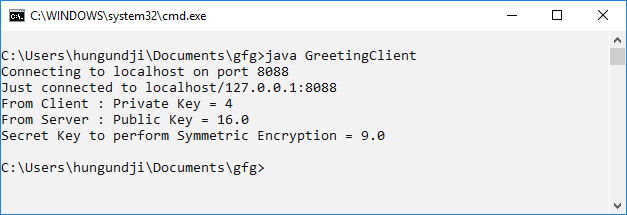GFG App
Open AppBrowser
Continue

# Java Implementation of Diffie-Hellman Algorithm between Client and Server

Program to implement Diffie-Hellman Algorithm in Client-Server Fashion. Prerequisite: Server Socket Programming, Diffie-Hellman algorithm The Diffie Hellman Algorithm is being used to establish a shared secret that can be used for secret communications while exchanging data over a public network. In the below program, the client will share the value of, and public key. Whereas, the server will accept the values and calculate its public keyand send it to the client. Both Client and Server will calculate the secret key for symmetric encryption by using the public key. Program 1: Server Program

## Java

 `import` `java.net.*;` `import` `java.io.*;`   `public` `class` `GreetingServer {` `    ``public` `static` `void` `main(String[] args) ``throws` `IOException` `    ``{` `        ``try` `{` `            ``int` `port = ``8088``;`   `            ``// Server Key` `            ``int` `b = ``3``;`   `            ``// Client p, g, and key` `            ``double` `clientP, clientG, clientA, B, Bdash;` `            ``String Bstr;`   `            ``// Established the Connection` `            ``ServerSocket serverSocket = ``new` `ServerSocket(port);` `            ``System.out.println("Waiting ``for` `client on port " + serverSocket.getLocalPort() + "...");` `            ``Socket server = serverSocket.accept();` `            ``System.out.println("Just connected to " + server.getRemoteSocketAddress());`   `            ``// Server's Private Key` `            ``System.out.println("From Server : Private Key = " + b);`   `            ``// Accepts the data from client` `            ``DataInputStream in = ``new` `DataInputStream(server.getInputStream());`   `            ``clientP = Integer.parseInt(in.readUTF()); ``// to accept p` `            ``System.out.println("From Client : P = " + clientP);`   `            ``clientG = Integer.parseInt(in.readUTF()); ``// to accept g` `            ``System.out.println("From Client : G = " + clientG);`   `            ``clientA = Double.parseDouble(in.readUTF()); ``// to accept A` `            ``System.out.println("From Client : Public Key = " + clientA);`   `            ``B = ((Math.pow(clientG, b)) % clientP); ``// calculation of B` `            ``Bstr = Double.toString(B);`   `            ``// Sends data to client` `            ``// Value of B` `            ``OutputStream outToclient = server.getOutputStream();` `            ``DataOutputStream out = ``new` `DataOutputStream(outToclient);`   `            ``out.writeUTF(Bstr); ``// Sending B`   `            ``Bdash = ((Math.pow(clientA, b)) % clientP); ``// calculation of Bdash`   `            ``System.out.println("Secret Key to perform Symmetric Encryption = "` `                               ``+ Bdash);` `            ``server.close();` `        ``}`   `        ``catch` `(SocketTimeoutException s) {` `            ``System.out.println("Socket timed out!");` `        ``}` `        ``catch` `(IOException e) {` `        ``}` `    ``}` `}`

Program 2: Client Program

## Java

 `import` `java.net.*;` `import` `java.io.*;`   `public` `class` `GreetingClient {` `    ``public` `static` `void` `main(String[] args)` `    ``{` `        ``try` `{` `            ``String pstr, gstr, Astr;` `            ``String serverName = "localhost";` `            ``int` `port = ``8088``;`   `            ``// Declare p, g, and Key of client` `            ``int` `p = ``23``;` `            ``int` `g = ``9``;` `            ``int` `a = ``4``;` `            ``double` `Adash, serverB;`   `            ``// Established the connection` `            ``System.out.println("Connecting to " + serverName` `                               ``+ " on port " + port);` `            ``Socket client = ``new` `Socket(serverName, port);` `            ``System.out.println("Just connected to "` `                               ``+ client.getRemoteSocketAddress());`   `            ``// Sends the data to client` `            ``OutputStream outToServer = client.getOutputStream();` `            ``DataOutputStream out = ``new` `DataOutputStream(outToServer);`   `            ``pstr = Integer.toString(p);` `            ``out.writeUTF(pstr); ``// Sending p`   `            ``gstr = Integer.toString(g);` `            ``out.writeUTF(gstr); ``// Sending g`   `            ``double` `A = ((Math.pow(g, a)) % p); ``// calculation of A` `            ``Astr = Double.toString(A);` `            ``out.writeUTF(Astr); ``// Sending A`   `            ``// Client's Private Key` `            ``System.out.println("From Client : Private Key = " + a);`   `            ``// Accepts the data` `            ``DataInputStream in = ``new` `DataInputStream(client.getInputStream());`   `            ``serverB = Double.parseDouble(in.readUTF());` `            ``System.out.println("From Server : Public Key = " + serverB);`   `            ``Adash = ((Math.pow(serverB, a)) % p); ``// calculation of Adash`   `            ``System.out.println("Secret Key to perform Symmetric Encryption = "` `                               ``+ Adash);` `            ``client.close();` `        ``}` `        ``catch` `(Exception e) {` `            ``e.printStackTrace();` `        ``}` `    ``}` `}`

Use javac to Compile the programs, and open two console/terminal to run the system Output: In the first console run the server program, it will wait for the client’s connection. As soon as client is connected results will popupIn the second console, run the client’s programI have provided a Java implementation of the Diffie-Hellman algorithm in my previous response. However, in terms of the advantages of using the Diffie-Hellman algorithm, here are some key benefits:

1. Secure key exchange: The Diffie-Hellman algorithm allows two parties to securely exchange a shared secret key over an insecure communication channel. This shared key can then be used for subsequent encryption and decryption of messages.
2. Resistance to attacks: The Diffie-Hellman algorithm is resistant to a variety of attacks, including man-in-the-middle attacks and eavesdropping attacks. This makes it a strong choice for securing communication channels.
3. Scalability: The Diffie-Hellman algorithm can be easily scaled to accommodate large numbers of parties. This makes it ideal for use in group communication scenarios.
4. Flexibility: The Diffie-Hellman algorithm can be used with a variety of cryptographic primitives, including symmetric-key encryption and digital signatures. This flexibility allows it to be used in a wide range of security applications.

Overall, the Diffie-Hellman algorithm provides a secure and efficient method for exchanging keys between two parties over an insecure communication channel. Its resistance to attacks, scalability, and flexibility make it a popular choice for securing communication channels in a variety of applications.

My Personal Notes arrow_drop_up